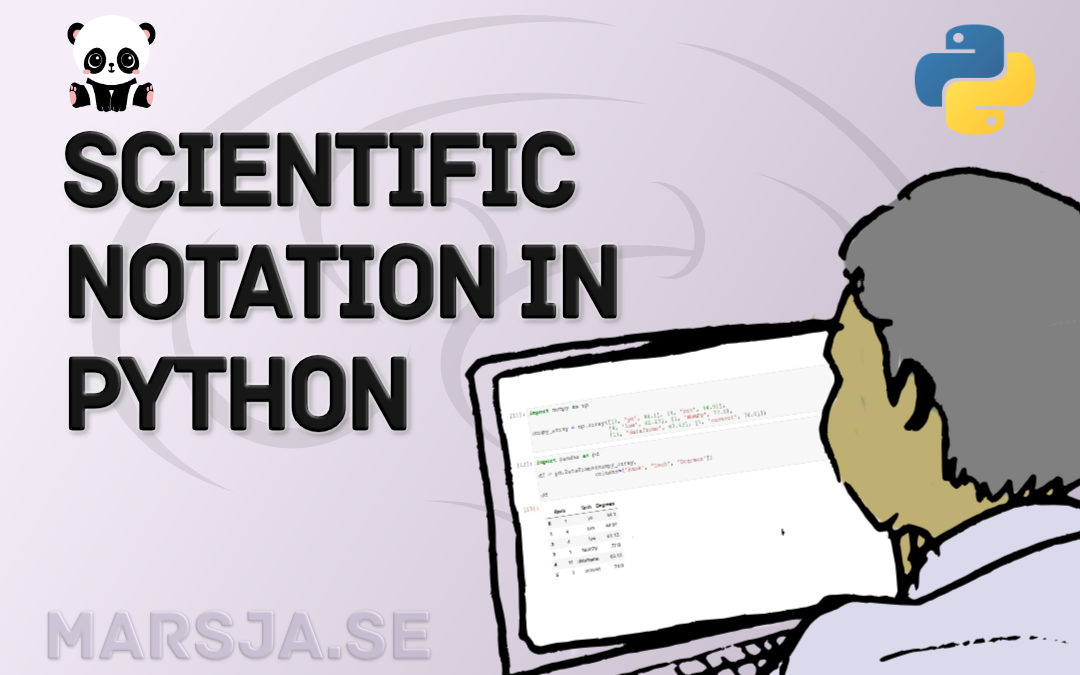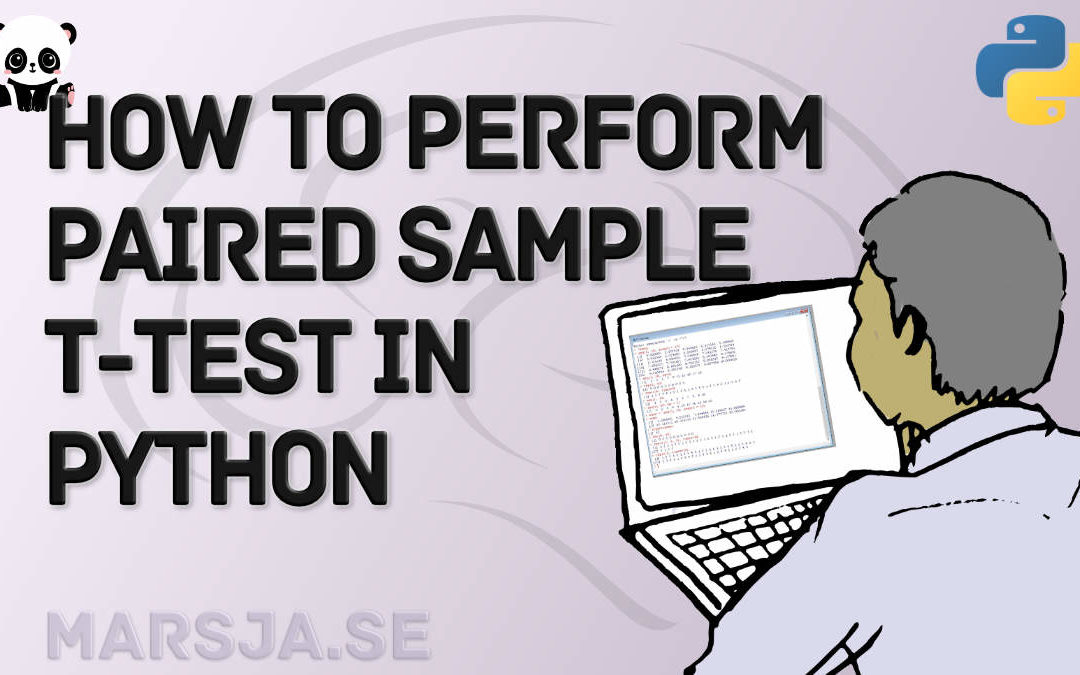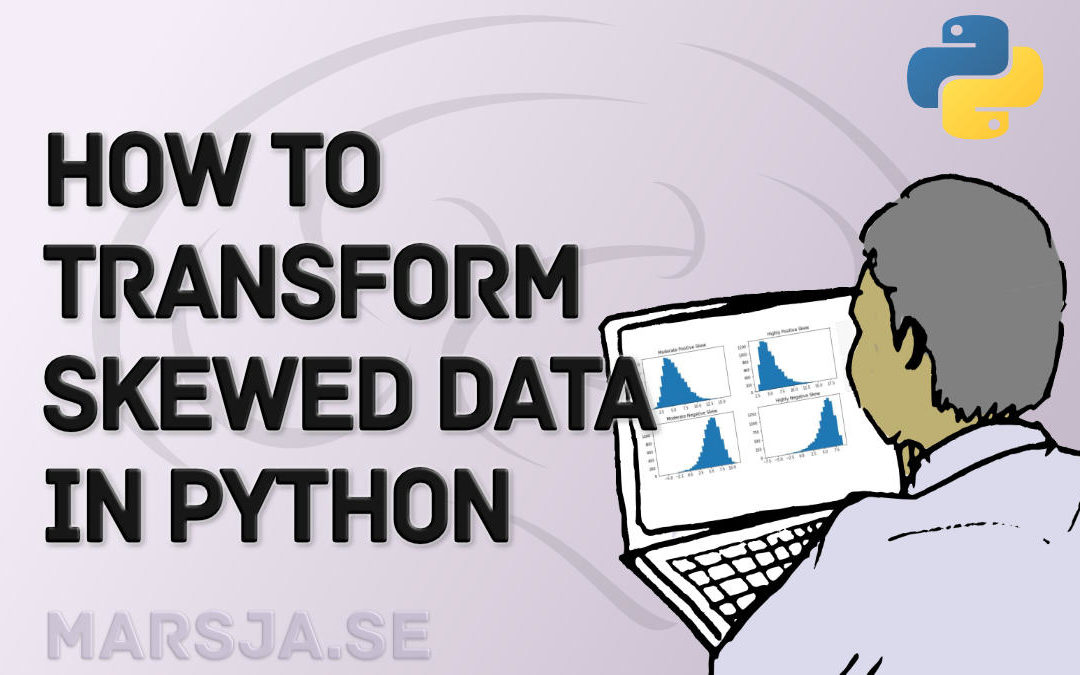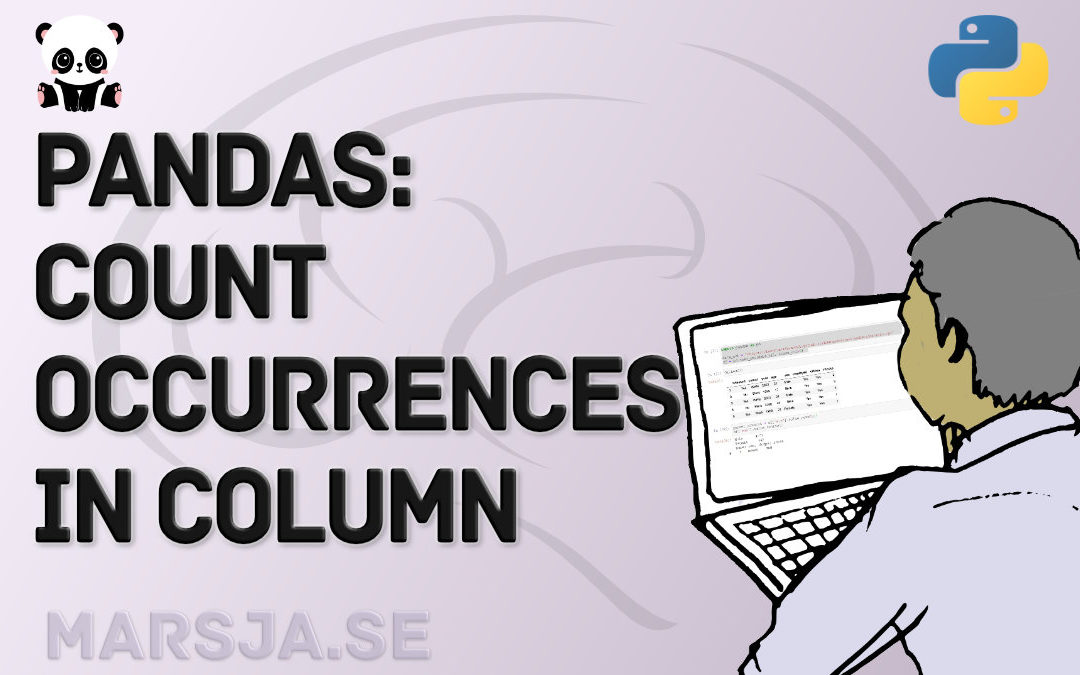## Python Scientific Notation & How to Suppress it in Pandas and NumPy

In Python, it is possible to print numbers in scientific notation using base functions as well as NumPy. Specifically, you will learn how to use Python to print very large or very small  (i.e., floating point) numbers in scientific notation using three different...## How to Make a Violin plot in Python using Matplotlib and Seaborn

In this Python data visualization tutorial, we are going to learn how to create a violin plot using Matplotlib and Seaborn. Now, there are several techniques for visualizing data (see the post 9 Data Visualization Techniques You Should Learn in Python for some...## How to use Python to Perform a Paired Sample T-test

In this Python data analysis tutorial, you will learn how to perform a paired sample t-test in Python. First, you will learn about this type of t-test (e.g. when to use it, the assumptions of the test). Second, you will learn how to check whether your data follow the...## How to use Square Root, log, & Box-Cox Transformation in Python

In this tutorial, related to data analysis in Python, you will learn how to deal with your data when it is not following the normal distribution. One way to deal with non-normal data is to transform your data. In this post, you will learn how to carry out Box-Cox,...## Levene’s & Bartlett’s Test of Equality (Homogeneity) of Variance in Python

In this Python tutorial, you will learn how to 1) perform Bartlett’s Test, and 2) Levene’s Test. Both are tests that are testing the assumption of equal variances. Equality of variances (also known as homogeneity of variance, and homoscedasticity) in population...## Pandas Count Occurrences in Column – i.e. Unique Values

In this Pandas tutorial, you are going to learn how to count occurrences in a column. There are occasions in data science when you need to know how many times a given value occurs. This can happen when you, for example, have a limited set of possible values that you...